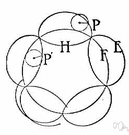hypocycloid

(redirected from Hypocycloids)
Also found in: Thesaurus, Encyclopedia.
hypocycloid
If a is the radius of a fixed circle and b is the radius of a smaller rotating circle, the parametric equations of the hypocycloid are x = (a - b) cos θ + b cos [(a - b) θ ]/b
y = (a - b) sin θ - b sin [( a - b) θ ]/b.

hy·po·cy·cloid

(hī′pō-sī′kloid′)
n.
The plane locus of a point fixed on a circle that rolls on the inside circumference of a fixed circle.

hypocycloid

(ˌhaɪpəˈsaɪklɔɪd)
n
(Mathematics) a curve described by a point on the circumference of a circle as the circle rolls around the inside of a fixed coplanar circle. Compare epicycloid, cycloid4

hy•po•cy•cloid

(ˌhaɪ pəˈsaɪ klɔɪd)

n.
a curve generated by the motion of a point on the circumference of a circle that rolls internally, without slipping, on a fixed circle.
[1835–45]
 Noun 1hypocycloid - a line generated by a point on a circle that rolls around inside another circleline roulette, roulette - a line generated by a point on one figure rolling around a second figure